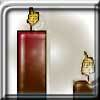#### You may also like### Burning Down

One night two candles were lit. Can you work out how long each candle was originally?### Percentage Unchanged

If the base of a rectangle is increased by 10% and the area is unchanged, by what percentage is the width decreased by ?### Digit Sum

What is the sum of all the digits in all the integers from one to one million?

# Rough Root

##### Age 14 to 16 ShortChallenge Level

Squaring the options
$\dfrac{10 000}{2012}\approx\dfrac{10000}{2000}=5$, so $\sqrt{\dfrac{10000}{2012}}\approx\sqrt5$
(We should really check how good this approximation is. See below (or click here) for comparison).

So one of the options should square to a number close to $5$. Squaring them gives:
$1.9^2=3.61$ which is too small
$2.2^2=4.84$ which is too small
$2.5^2=6.25$ which is too big
so $2.7^2$ will definitely be too big.

$2.2$ is too small and $2.5$ is too big. To find which is closer to $\sqrt5$, test a number in between.
If $2.3$ is too big, then we will know that $\sqrt5$ is between $2.2$ and $2.3$, so it is definitely closer to $2.2$ than to $2.5$.

$2.3^2=2.3\times2+2.3\times0.3=4.6+0.69=5.29$, which is too big.

So $2.2$ is the closest.

Estimating the square root
$10 000=100^2$

$\dfrac{100}?\times\dfrac{100}?=\dfrac{10000}{?\times?}$ where $?\times?$ is close to $2012$

$40^2=1600$ and $50^2=2500$. Try $45^2=2025$

$\therefore\dfrac{100}{45}\times\dfrac{100}{45}=\dfrac{10000}{2025}\approx\dfrac{10000}{2012}$

(We should really check how close this approximation is. See below (or click here) for comparison)

So $\sqrt{\dfrac{10000}{2012}}\approx\dfrac{100}{45}=\dfrac{20}{9}=2.\dot2$

So $2.2$ is the closest.

How close are our approximations?
The difference between $\dfrac{10 000}{2012}$ and $5$ is: \begin{align}\frac{10000}{2000}-\frac{10000}{2012}&=1000\left(\frac{1}{2000}-\frac1{2012}\right)\\ &=10000\left(\frac{2012-2000}{2000\times2012}\right)\\ &=\frac{10000\times12}{2000\times2012}\\ &=\frac{10\times6}{2012}=\frac{30}{1006}<\frac{30}{1000}=0.03\end{align} So really we were looking for a number whose square was somewhere between $5$ and $5.03$. Squaring the options gave results far less precise than this, so this approximation was good enough for this situation.

The difference between $\dfrac{10 000}{2012}$ and $\dfrac{10000}{2025}$ is: \begin{align}\dfrac{10 000}{2012}-\dfrac{10000}{2025}&=10000\left(\dfrac1{2012}-\dfrac{1}{2025}\right)\\ &=10000\left(\frac{2025-2012}{2012\times2025}\right)\\ &=\frac{10000\times13}{2025\times2012}<\frac{10000\times13}{2000\times2000}=\frac{13}{2\times200}<\frac{16}{400}=\frac4{100}=0.04\end{align}
So the square of $2.\dot2$ is too large, by up to $0.04$.
We can write this as $2.\dot2^2-c^2<0.04$, where $c$ is the exact value of $\sqrt{\dfrac{10000}{2012}}$
We can factorise $2.\dot2^2-c^2$ as the difference of two squares:
$2.\dot2^2-c^2=(2.\dot2+c)(2.\dot2-c)$, so $(2.\dot2+c)(2.\dot2-c)<0.04$

$2.\dot2-c$ must be very close to $0$, because the product is close to $0$, and $(2.\dot2+c)$ is not particularly close to $0$. This is good, because if $2.\dot2$ is a good approximation, then $2.\dot2-c$ is close to $0$.

In fact, $(2.\dot2+c)$ must be more than $2$, since $c$ is positive. So $2.\dot2-c$ must less than $0.02$, to give a product of less than $0.04$ (since $2\times0.02=0.04$).

So $2.\dot2$ is an over-estimation by less than $0.02$, which means that the true value of $c$ is somewhere between $2.\dot2-0.02=2.20\dot2$ and $2.\dot2$. So $2.2$ is defiitely the closest of the options. Our approximation was actually far better than the question required.
You can find more short problems, arranged by curriculum topic, in our short problems collection.